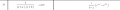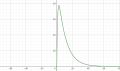# Bode plot / spectrum: How to determin time function?

#### LvW

Joined Jun 13, 2013
1,646
What do you mean with "time function"?
The output voltage vs. time is a function of the input voltage.
Perhaps you mean "step response"?

#### Jiwoo

Joined Feb 6, 2023
19
@LvW Yes exactly! How do I determine the shape and the period time τ or T?

#### LvW

Joined Jun 13, 2013
1,646
Well, there is a certain relaton between the frequency domain and the time domain.
In detail: The step response g(t) is the Laplace transform of the system function H(s) - multiplied with "s".
However, there is a simpler way to find g(t):
* Derive the system function H(s) from the Bode plot. In your case, I can identify two poles and the DC gain.
Therefore, it is easy to write down the function H(s).
* From this 2nd-order function you can derive the quality factor Qp (pole-Q) and the characteristic frequency wo.
* This is all you need to get a rough information about the form of the step response g(t).
(Remember: There is a relation between the pole-Q (frequency domain) and the overshoot (time domain).

Added: The abov applies to a 2nd-order function with a complex pole-pair. In case of two real poles there are some other rules which help to find the step response as a function of the two time constants

Last edited:

#### Jiwoo

Joined Feb 6, 2023
19
@LvW Well K seems to be 100 --> H(s) = 100/(s+0,1)+(s+1). According to this formula g(t9 is determined as following:I used the formula but I got the wrong plot.The time function must have the shape of trapezoid or rectangle. What did I do wrong?

#### LvW

Joined Jun 13, 2013
1,646
You are mentioning "the time function". Please, be exact - do you mean input or output?
Please, show the units for the axis of the plot - otherwise, one cannot comment on this.
More than that - the "edges" (poles) in the bode plot are given in terms of frequency - however, in the transfer function they must appear as w=2*Pi*f.

#### RoofSheep

Joined Mar 7, 2023
22
Why do you think the time-domain function must be a trapezoid or a rectangle. Calculate the Laplace transforms of the two and see if they correspond to the shape of your Bode plot.

#### MrAl

Joined Jun 17, 2014
10,087
Hey,

I have this bode plot given and I have to determin the time function. The amplitude is 40 dBuVs. The time function will probably be a rectangle, but idk how to determine the time constant τ. Is it like T = 1/f? Thank you very much

View attachment 287008
That looks like a low pass filter, is that what it is?

#### MrAl

Joined Jun 17, 2014
10,087
Well, there is a certain relaton between the frequency domain and the time domain.
In detail: The step response g(t) is the Laplace transform of the system function H(s) - multiplied with "s".
However, there is a simpler way to find g(t):
* Derive the system function H(s) from the Bode plot. In your case, I can identify two poles and the DC gain.
Therefore, it is easy to write down the function H(s).
* From this 2nd-order function you can derive the quality factor Qp (pole-Q) and the characteristic frequency wo.
* This is all you need to get a rough information about the form of the step response g(t).
(Remember: There is a relation between the pole-Q (frequency domain) and the overshoot (time domain).

Added: The abov applies to a 2nd-order function with a complex pole-pair. In case of two real poles there are some other rules which help to find the step response as a function of the two time constants
Hi,

Sorry but i think you meant the step response of the system is the function H(s) divided by "s" not multiplied. Then of course you use the Inverse Laplace Transform to get the time domain response.

Sorry again though, but even that is not exactly correct. The step response is actually the system function H(s) with the input source (a constant value) divided by "s". To divide H(s) by "s" is a common way to do it but it only works if H(s) happened to be in the form of a unit F(s) that was multiplied by the input source, in the form Vin*F(s).
So the correct form to use is:
[Vin/s]*F(s)

This is not exactly the same as [Vin*F(s)]/s although that commonly works with a lot of transfer functions.

Last edited:

#### MrAl

Joined Jun 17, 2014
10,087
@LvW Well K seems to be 100 --> H(s) = 100/(s+0,1)+(s+1). According to this formula g(t9 is determined as following:
View attachment 287109
I used the formula but I got the wrong plot.
View attachment 287110
The time function must have the shape of trapezoid or rectangle. What did I do wrong?
It could be that you need to use a function that is more damped than what you are using. That means you may have the wrong values for a and b.
Look up what the "damping coefficient" is in a second order system.
You will probably get a time function that looks like your frequency domain function graph only in reverse, with the response starting out at zero then climbing then leveling off.

#### LvW

Joined Jun 13, 2013
1,646
Sorry but i think you meant the step response of the system is the function H(s) divided by "s" not multiplied. Then of course you use the Inverse Laplace Transform to get the time domain response.
Yes - you are right. Thank you.
What I wrote was just the opposite: How to derive the transfer function H(s) from the step response.
When we are looking for the step response, of course, we have to apply the inverse Laplace transform to the expression H(s)/s .

#### MrAl

Joined Jun 17, 2014
10,087
Yes - you are right. Thank you.
What I wrote was just the opposite: How to derive the transfer function H(s) from the step response.
When we are looking for the step response, of course, we have to apply the inverse Laplace transform to the expression H(s)/s .
Hi,

Oh yes, and i only brought up the second point because sometimes the original H(s) is in the form of:
Vout(s)=Vin*A+B, where B is not a function of Vin